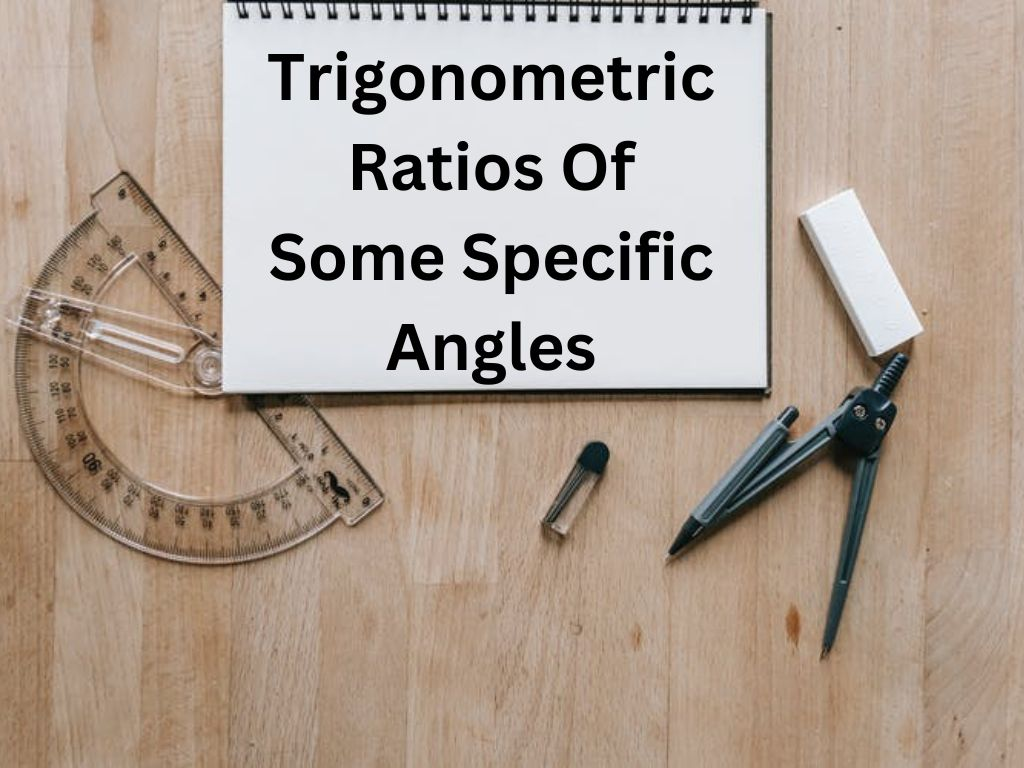# Trigonometric Ratios of Some Specific Angles Class 10th

In Trigonometry, For all the trigonometric ratios we will find their values for some specific angles 0°, 30°, 45°, 60°, and 90°.

### Trigonometric Ratios of Angle 0°

Let △ABC be a right-angled triangle in which ∠B is the right angle and ∠C is an acute angle ϴ. If the angle ϴ becomes 0° then line segment AC(Hypotenuse) will coincide with line segment BC(Base) and line segment AB(Perpendicular) will become 0. it means AB = 0 and AC = BC.

Thus, the values of trigonometric ratios for angle 0°

### Trigonometric Ratios of Angles 30° and 60°

Let △ABC be an equilateral triangle with each side 2a. Therefore AB=BC=CA=2a and ∠A=∠B=∠C=60°. AD is perpendicular on side BC from vertex A (AD ⊥ BC).

In △ABD and △ACD,

AB = AC = 2a

By RHS criterion, △ABD ≅ △ACD

Therefore, BD = CD = a and ∠BAD = ∠CAD = 30° (by CPCT)

In a right-angled triangle ABD

AB = 2a, BD = a

AD2 = AB2 – BD2 (by Pythagoras theorem)

AD2 = (2a)2 – (a)2 = 4a2 – a2

### Trigonometric Ratios of Angle 45°

Let △ABC be a right-angled triangle. In which ∠B is the right angle and ∠C = 45°.

Now ∠A + ∠B + ∠C = 180°

∠A + 90° + 45° = 180°

∠A = 180° – 135°

∠A = 45°

Since ∠A = ∠C

Therefore, AB = BC (opposite sides of equal angles are equal)

Let AB = BC = a

Then AC2 = AB2 + BC2 (by Pythagoras theorem)

AC2 = (a)2 + (a)2 = a2 + a2

AC = √2a2 = a√2

Thus, the values of trigonometric ratios for angle 45°

### Trigonometric Ratios of Angle 90°

Let △ABC be a right-angled triangle in which ∠B is the right angle and ∠C is an acute angle ϴ. If the angle ϴ becomes 90° then line segment AC(Hypotenuse) will coincide with line segment AB(Perpendicular) and line segment BC(Base) will become 0. it means BC = 0 and AC = AB.

Thus, the values of trigonometric ratios for angle 90°

From all the above values if we make a table so all these values will be remembered easily.

#### Steps to Remember the Table Quickly –

1) First of all, we have to write numbers 0, 1, 2, 3, and 4 for the angles 0°, 30°, 45°, 60°, and 90° respectively.

2) After that we shall divide each number by 4 as follows

3) Now we have the numbers 0, 1/4, 1/2, 3/4, and 1. We shall take the square root of these numbers.

These values are for sin ϴ.

4) By writing these values in the reverse order we shall get the values for cos ϴ.

5) For tan ϴ, we shall divide the values of sin ϴ by values of cos ϴ because tan ϴ = sin ϴ/cos ϴ.

6) Now we shall take the reciprocal of the values of sin ϴ to get the values of cosec ϴ because cosec ϴ = 1/sin ϴ.

7) For sec ϴ, we shall write the values of cosec ϴ in the reverse order.

8) For cot ϴ, we shall take the reciprocal of the values of tan ϴ because cot ϴ = cos ϴ/sin ϴ.

#### Examples –

Example – 1) find the value of sin 60° cos 30° – sin 30° cos 60°.

Solution – sin 60° cos 30° – sin 30° cos 60°

(√3/2)⨯(√3/2) – (1/2)⨯(1/2) = 3/4 – 1/4 = 3-1/4 = 2/4 = 1/2 Ans.

Example – 2) find the value of tan2 45° + sin2 30° – cos2 60°

Solution – tan2 45° + sin2 30° – cos2 60°

(1)2 + (1/2)2 – (1/2)2 = 1 + 1/4 – 1/4 = 1 Ans.

Trigonometric Ratios of Some Specific Angles Class 10th in Hindi

More About Trigonometric Ratios of Some Specific Angles

Rate this post